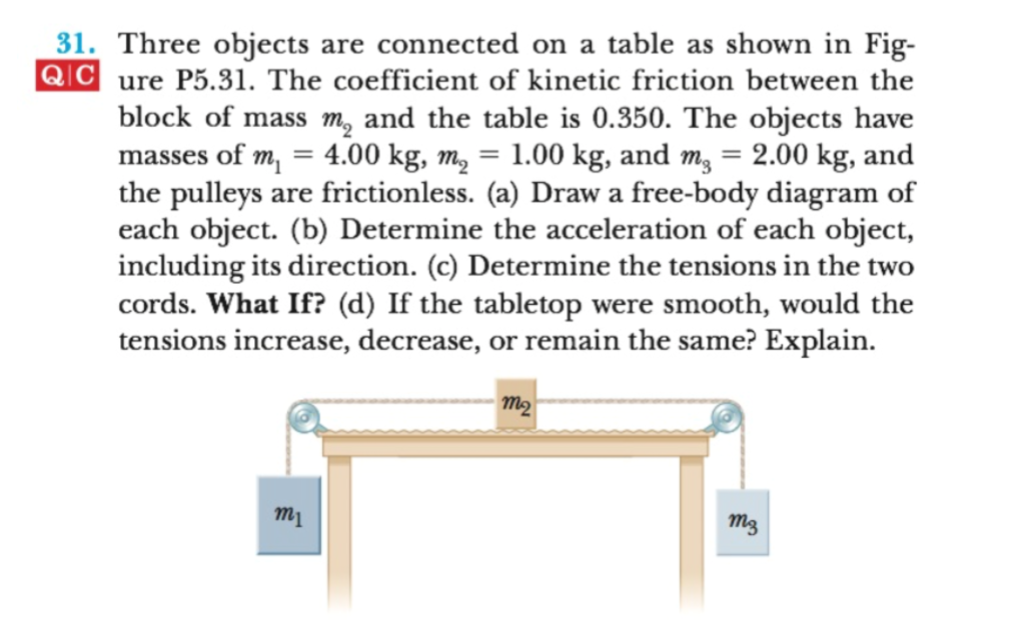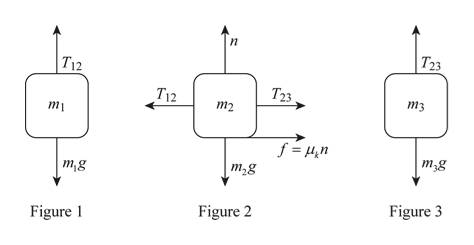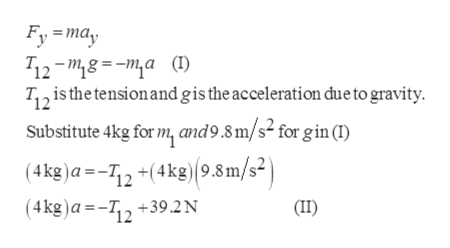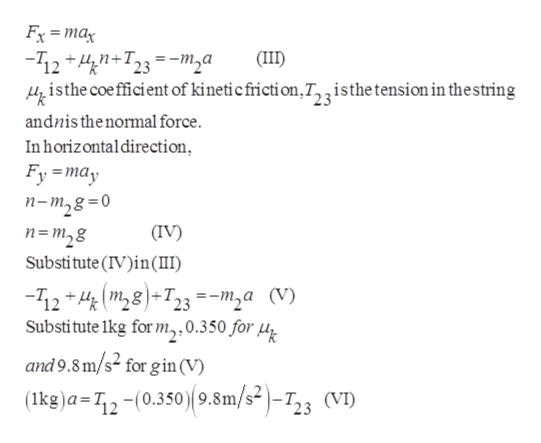31. Three objects are connected on a table as shown in Fig-QCure P5.31. The coefficient of kinetic friction between theblock of mass m and the table is 0.350. The objects havemasses of m 4.00 kg, m 1.00 kg, and m 2.00 kg, andthe pulleys are frictionless. (a) Draw a free-body diagram ofeach object. (b) Determine the acceleration of each object,including its direction. (c) Determine the tensions in the twocords. What If? (d) If the tabletop were smooth, would thetensions increase, decrease, or remain the same? Explainтэтimg

Questionhelp_outlineImage Transcriptionclose31. Three objects are connected on a table as shown in Fig- QCure P5.31. The coefficient of kinetic friction between the block of mass m and the table is 0.350. The objects have masses of m 4.00 kg, m 1.00 kg, and m 2.00 kg, and the pulleys are frictionless. (a) Draw a free-body diagram of each object. (b) Determine the acceleration of each object, including its direction. (c) Determine the tensions in the two cords. What If? (d) If the tabletop were smooth, would the tensions increase, decrease, or remain the same? Explain тэ тi mg fullscreen
Step 1

a)

The mass of block 1 is 4kg, mass of block 2 is 1kg, the mass of block 3 is 2kg and the coefficient of kinetic friction between mass and the table is 0.350.

The free body diagram of each of the block is given belowhelp_outlineImage Transcriptioncloseп Тz3 T12 Та3. T12 тз т2 ту YΕμη m,g т,g тg Figure 3 Figure 2 Figure fullscreen
Step 2

b)

Acceleration of m1 is –a ĵ, the acceleration of m2 is –a ĵ and the acceleration of m3 is +a ĵ. The magnitude of acceleration for all the three masses is a.

Write the expression for the net force on blockhelp_outlineImage TranscriptioncloseFy may 12-"8a(1) T2 is the tension and gis the acceleration due to gravity Substitute 4kg for m, and9.8 m/s2 for gin (I) (4kg)a=12+(4kg)(9.8m/s2 (4kg)a=-T2+39.2N (II) fullscreen
Step 3

Write the expression for ne...help_outlineImage TranscriptioncloseFx ma 12 +T23ma isthe coe fficient of kineticfricti on,T,,isthetension in thestring (II andnisthenormal force. In horizontal direction, Fy=may п-т,8 3D0 п-т,8 Substitute (IV)in(III) (IV) -12 (m28T23=-m,a (V) Substitute 1kg for m,, 0.350 for u and 9.8m/s2 for gin (V) (kg)a=12-(0.350) 9.8m/s2)-723 (VI) fullscreen

Want to see the full answer?

See Solution

Want to see this answer and more?

Our solutions are written by experts, many with advanced degrees, and available 24/7

See Solution
Tagged in

Kinematics